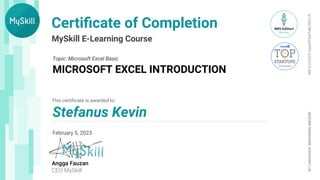Successfully reported this slideshow.

# Microsoft excel Introduction×

# Microsoft excel Introduction

Learned :

1. Cell reference
2. Relative and absolute
3. Shortcut
4. Formula and functions
5. Options
6. Cells to calculation practice using microsoft excel

For example using (=SUM) and Shortcut Ctrl and Shift panel button for a faster calculation and paste.

Learned :

1. Cell reference
2. Relative and absolute
3. Shortcut
4. Formula and functions
5. Options
6. Cells to calculation practice using microsoft excel

For example using (=SUM) and Shortcut Ctrl and Shift panel button for a faster calculation and paste.